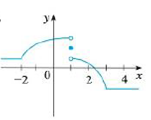Chapter 2.8, Problem 44E### Single Variable Calculus: Early Tr...

8th Edition
James Stewart
ISBN: 9781305270343

#### Solutions

Chapter
Section### Single Variable Calculus: Early Tr...

8th Edition
James Stewart
ISBN: 9781305270343
Textbook Problem

# The graph of f is given. State, with reasons, the numbers at which f is not differentiable.To determine

To state: The graph of the function f is not differentiable at which the numbers.

Explanation

Note:

The function f is not differentiable at the point a, then it must be satisfied any of the following conditions.

(i) The function f is discontinuous at the point a.

(ii) The function f has a corner point at the point a.

(iii) The function f has a vertical tangent at the point a.

From the graph of f, it is observed that f has a corner points at x=2 and x=3

### Still sussing out bartleby?

Check out a sample textbook solution.

See a sample solution

#### The Solution to Your Study Problems

Bartleby provides explanations to thousands of textbook problems written by our experts, many with advanced degrees!

Get Started

#### Evaluate the expression sin Exercises 116. 323

Finite Mathematics and Applied Calculus (MindTap Course List)

#### True or False: ln(a + b) = ln a + ln b.

Study Guide for Stewart's Single Variable Calculus: Early Transcendentals, 8th

#### Write a research report using APA style.

Research Methods for the Behavioral Sciences (MindTap Course List)